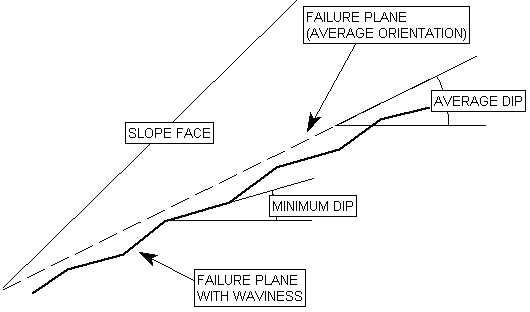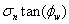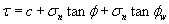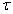Join one of our upcoming courses taking place around the world! Explore now

# Failure Plane

The failure plane is the surface along which sliding of a block or wedge occurs.

## Angle

This value refers to the dip of the failure or sliding plane in a model.

## Waviness

Waviness is a parameter that can be included in calculations of the shear strength of the failure plane, for any of the strength models used in RocPlane. It accounts for the waviness (undulations) of the failure plane surface, observed over distances on the order of 1 m to 10 m [Miller, 1988], and has the effect of INCREASING the shear strength of the failure plane, as described below.

The waviness angle is equal to the AVERAGE dip of the failure plane, minus the MINIMUM dip of the failure plane. This is illustrated in the figure below.

The waviness angle can be determined by taking a number of failure plane dip measurements, calculating the average dip of the failure plane and subtracting the minimum dip. For example, if the AVERAGE failure plane dip = 30 degrees, and the MINIMUM failure plane dip = 25 degrees, then the waviness angle = 5 degrees.

Waviness Angle = AVERAGE DIP – MINIMUM DIP of failure plane### EFFECT OF WAVINESS ANGLE ON SHEAR STRENGTH

A non-zero waviness angle, will INCREASE the effective shear strength of the failure plane, as described below.

The implementation of the waviness angle in the factor of safety equation is very simple. The INCREASE in the failure plane shear strength, due to waviness, is simply equal to:where:= normal stress on the failure plane= waviness angle

For example, if you are using the Mohr-Coulomb failure criterion, and you have specified a waviness angle greater than zero, then the shear strength of the failure plane is given by:where:= shear strength= cohesion= friction angle

As you can see, the waviness angle is implemented in the same way as the Mohr-Coulomb friction angle. The increased shear strength is proportional to the normal stress and the tangent of the waviness angle. The effect of the waviness angle on shear strength is the same for all strength models used in RocPlane. The failure plane shear strength is simply INCREASED by the amount:regardless of which strength model you are using in RocPlane. For a description of the strength models which are available in RocPlane, see the Strength topic.